# C if if-else if-else-if Statement

In this tutorial, you will learn all about if, if-else, if-else-if Statement in C programming with example programs. Let's start with if statement in C.

## C if statement

Here is the general form of the if statement:

```if(expression)
{
statement;
}
else
{
statement;
}```

Here statement may consist of a single statement or multiple statements (block of statements), or nothing (in case of empty statements). And the else clause is optional.

Here, if the expression evaluates to true i.e., anything other than 0, then the targeted statement or the block of statements of if will be executed, otherwise the targeted statement or the block of statements of else will be executed if present.

Note - Only the code associated with the if or the code associated with the else executes, never both.

### C if Statement Example

The following C program generate a random number using rand() function of <stdlib.h>. This program ask to guess and enter any number to match with the generated random number. If the entered number and the random number will become equal then the program will print "You are a random number generator", otherwise it will not print anything and exit the program:

```/* C Decision Making - The if Statement Example Program */

#include<stdio.h>
#include<conio.h>
#include<stdlib.h>

void main()
{
int magic, guess;
clrscr();

magic = rand();     // generate the random number and initialize to magic
printf("Guess the number : ");
scanf("%d", &guess);

if(guess==magic)
{
printf("You are a random number generator");
}

getch();
}```

Here is the two output of the above C program, the first one, if the entered number matched by the the generated number and the other one, if the entered number is not matched by the generated number: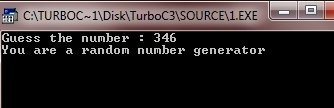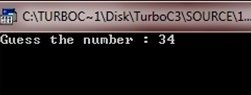Now in the next C program, there is a uses of else along with if to print the message if the entered number will not match to the generated random number. Here if the entered number will match to the generated random number, then it will print "You are a random number generator", otherwise it will print "You are not a random number generator" using the else clause:

```/* C Decision Making Example Program */

#include<stdio.h>
#include<conio.h>
#include<stdlib.h>

void main()
{
int magic, guess;
clrscr();

magic = rand();
printf("Guess a number : ");
scanf("%d", &guess);

if(guess==magic)
{
printf("You are a random number generator");
}
else
{
printf("You are not a random number generator");
}

getch();
}```

Here is the two sample run of the above C program: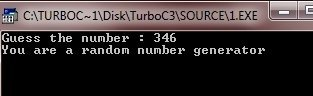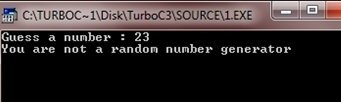## C Nested ifs Statement

A nested if is an if that is the target of another if or the else or the if...else. Here is the general form of the nested ifs Statement:

```if(expression1)
{
if(expression2)
{
statement;
}
if(expression3)
{
statement;
}
else
{
statement;
}
}
else
{
statement;
}```

Here is one more general form of nested ifs statement:

```if(a)
{
if(b)
{
if(c)
{
statement;
}
else
{
statement;
}
}
if(g)
{
statement;
}
else
{
statement;
}
}
else
{
if(k)
{
if(l)
{
statement;
}
if(m)
{
statement;
}
else
{
statement;
}
}
else
{
statement;
}
}```

### Evaluation of if Statement in C

The rule to evaluate the expression of nested ifs is same in any case, either it will be complicated or simple. First the if statement will evaluate, if the expression inside the bracket of if will return true then its targeted statement present inside the if will be executed, otherwise else's targeted statement will evaluate. Now inside the if statement if any other if statement present then it evaluate first, if the expression of this if will return true then the targeted statements inside this if statement will be executed, otherwise else's statement will be executed and so on.

### C Nested ifs Statement Example

Following C program shows the uses of simple nested ifs :

```/* C Decision Making - Nested if Statement Example */

#include<stdio.h>
#include<conio.h>
void main()
{
int mark;
clrscr();

printf("Enter marks in C Programming (out of 100) : ");
scanf("%d", &mark);

if(mark==100)
{
printf("Congrats..!!..You are a professional C programmer..!!");
}
else
{
if(mark>60)
{
printf("You are passed..!!");
}
else
{
printf("You are failed..!!");
}
}

getch();
}```

Here is the three sample run of the above C program.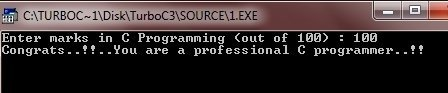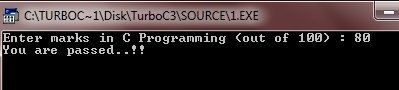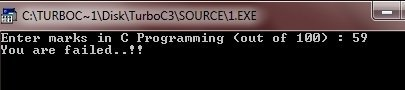Now here this C program have simple but complicated nested ifs statement:

```/* C Decision Making - Nested ifs Example Program */

#include<stdio.h>
#include<conio.h>
void main()
{
int mark;
clrscr();

printf("Enter marks obtained in C (out of 100) : ");
scanf("%d", &mark);
if(mark==100)
{
printf("Congrats..!!..You are a professional C programmer..!!");
getch();
exit(1);
}
if(mark>60 && mark<100)
{
if(mark>95 && mark<100)
{
if(mark==99)
{
printf("You are missed with 1 marks from professional C programmer");
getch();
exit(2);
}
else
{
printf("You are near to professional C programmer");
getch();
exit(3);
}
}
if(mark>90 && mark<96)
{
if(mark==95)
{
printf("You are an expert C programmer..!!");
getch();
exit(4);
}
else
{
printf("You are near to expert C programmer");
getch();
exit(5);
}
}
else
{
if(mark>85 && mark<91)
{
if(mark==90)
{
printf("You are missed with 5 marks from expert C programmer");
getch();
exit(6);
}
else
{
printf("You are an advance C programmer..!!");
getch();
exit(7);
}
}
if(mark>80 && mark<86)
{
printf("You are near to advance C programmer");
getch();
exit(8);
}
else
{
printf("You are just passed");
getch();
exit(9);
}

}
}
else
{
printf("You are failed");
getch();
exit(10);
}

getch();
}```

Here is all the desirable output produced by the above C program. In other word, here is the 10 output showing all the printf() statement one by one. Just look at these: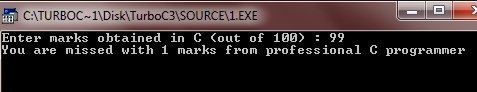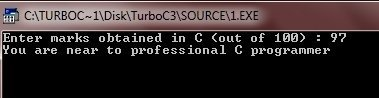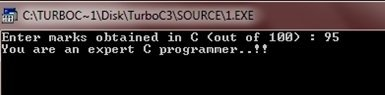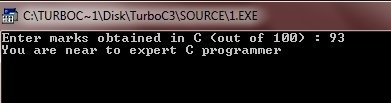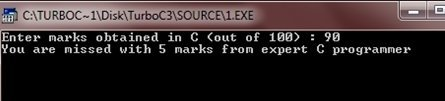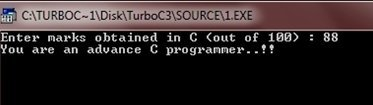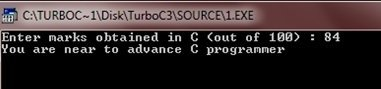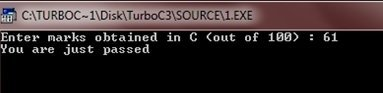The if-else-if ladder is a common programming construct. The if-else-if ladder is sometimes called as the if-else-if staircase because of its appearance. Here is the general form of the if-else-if ladder :

```if(expression)
{
statement;
}
else if(expression)
{
statement;
}
else if(expression)
{
statement;
}
.
.
.
else
{
statement;
}```

Following C program illustrates the if-else-if ladder:

```#include<stdio.h>
#include<conio.h>
void main()
{
int num;
clrscr();

printf("Enter a number (from 10-100) : ");
scanf("%d",&num);

if(num==100)
{
printf("You entered 100");
}
else if(num>90 && num<100)
{
printf("Its too high..!!\n");
printf("You entered %d", num);
}
else if(num>80 && num<90)
{
printf("Its high..!!\n");
printf("You entered %d", num);
}
else if(num<20 && num>10)
{
printf("Its too low..!!\n");
printf("You entered %d", num);
}
else if(num<30 && num>20)
{
printf("Its low..!!\n");
printf("You entered %d", num);
}
else
{
printf("You entered %d", num);
}

getch();
}```

Here is the 3 sample output of the above C program: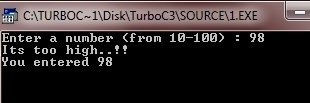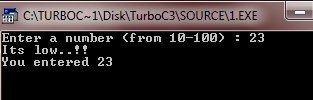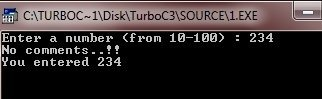### More Examples

Here are the list of some more examples that you can go for:

Tools
Calculator